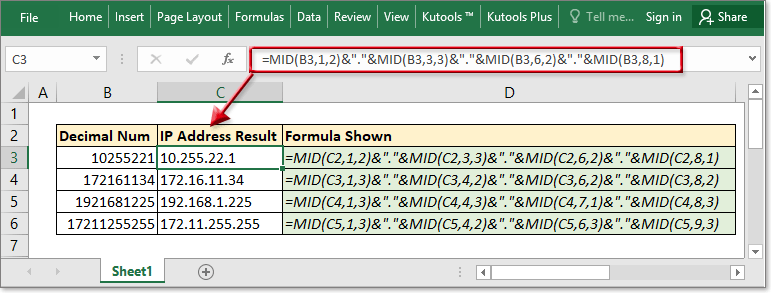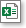Note: The other languages of the website are Google-translated. Back to English

## Convert decimal number to IP address

If you have a string of decimal numbers such as 192168421 that you need to convert to an IP address as192.168.42.1, how can you quickly solve this job with an Excel formula？### Convert decimal number to IP address with formula

Formula syntax

 =MID(number,1,num_of_digit)&"."&MID(number,start_position2,num_of_digit)&"."&MID(number,start_position3,num_of_digit)&"."&MID(number,start_position4,num_of_digit)

Arguments

 Number: Required, the decimal number that you want to convert to an IP address. 1: Required, it indicates to extract start from the first letter. Num_of_digit: Required, the number of digits needed to be extracted. Start_position2: Required, for the second part of the IP address, the position you want to extract numbers from. Start_position3: Required, for the third part of the IP address, the position you want to extract numbers from. Start_position4: Required, for the fourth part of the IP address, the position you want to extract numbers from.

Here are some examples in below table for you better understanding the formula.

 Decimal IP Address Formula 10255211 10.255.22.11 =MID(C2,1,2)&"."&MID(C2,3,3)&"."&MID(C2,6,2)&"."&MID(C2,8,1) 172161134 172.16.11.34 =MID(C3,1,3)&"."&MID(C3,4,2)&"."&MID(C3,6,2)&"."&MID(C3,8,2) 1921681225 192.168.1.225 =MID(C4,1,3)&"."&MID(C4,4,3)&"."&MID(C4,7,1)&"."&MID(C4,8,3) 17211255255 172.11.255.255 =MID(C5,1,3)&"."&MID(C5,4,2)&"."&MID(C5,6,3)&"."&MID(C5,9,3)

### Sample FileClick to download sample file

#### Relative Functions

• Excel DECIMAL function
The DECIMAL function converts a text representation of a number in a base into its equivalent decimal number. Take a instance, 11 is a text representation of 3 in base 2, using the formula =DECIMAL(11,2) convert 11 into decimal number 3.

### The Best Office Productivity Tools

#### Kutools for Excel - Helps You To Stand Out From Crowd

Would you like to complete your daily work quickly and perfectly? Kutools for Excel brings 300 powerful advanced features (Combine workbooks, sum by color, split cell contents, convert date, and so on...) and save 80% time for you.

• Designed for 1500 work scenarios, helps you solve 80% Excel problems.
• Reduce thousands of keyboard and mouse clicks every day, relieve your tired eyes and hands.
• Become an Excel expert in 3 minutes. No longer need to remember any painful formulas and VBA codes.
• 30-day unlimited free trial. 60-day money back guarantee. Free upgrade and support for 2 years.#### Office Tab - Enable Tabbed Reading and Editing in Microsoft Office (include Excel)

• One second to switch between dozens of open documents!
• Reduce hundreds of mouse clicks for you every day, say goodbye to mouse hand.
• Increases your productivity by 50% when viewing and editing multiple documents.
• Brings Efficient Tabs to Office (include Excel), Just Like Chrome, Firefox, And New Internet Explorer.No ratings yet. Be the first to rate!
This comment was minimized by the moderator on the site
These formulas and examples are incorrect. IP Addresses will never be represented as all of their digits with the decimal points removed. That would be a useless number. If you did that, there would be no way to tell if 10255221 was supposed to be 10.255.22.1 or 102.55.22.1 or 102.55.2.21? These are all valid IP addresses.

Dotted decimal notation (IP addresses) is just another form of notation, like binary or hexadecimal. It is 4, 8-bit, binary numbers, written in decimal notation, separated by decimal points. You can convert the numbers from one form to the other.

For example:
10.255.22.1 = 00001010.11111111.00010110.00000001 = 0a.ff.16.01 (0x0aff1601)
00001010 = 10 = 0xa
11111111 = 255 = 0xff
00010110 = 22 = 0x16
00000001 = 1 = 0x1
00001010111111110001011000000001 = 184489473 = 0xaff1601 = 10.255.22.1

In decimal notation, 10.255.22.1 = 184489473.
But 10.255.22.1 DOES NOT = 10255221. Ever.
There are no comments posted here yet